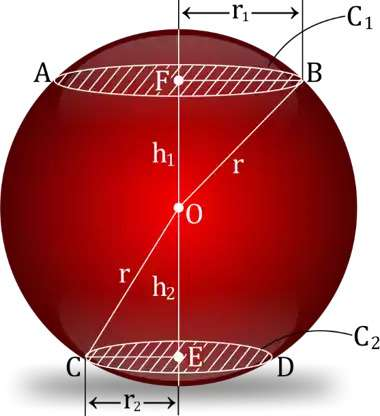Question of The Day30-01-2020

A sphere of radius r cm is cut into three parts by two parallel planes such that the total surface area is increased by 2πr2 due to the formation of new figures. One cut is at height h1, while other cut is at height h2 from the center. If the ratio of the heights h1 and h2 is 1 : 2, then what will be the value of h2 in terms of radius of the sphere?

Correct Answer : b ) $$\frac{2}{\sqrt{5}}\: r$$

Explanation :

Depicting the given information in the figure, we getAccording to the question, the total surface area of the resulting figures is increased by 2πr2. As increase in the area can only be because of addition of the new circular faces created because of the two cuts, therefore,

Increase in Total surface area of Sphere = 2* (Area of C1 + Area of C2)

2πr2 = 2πr12 + 2πr22

r12 + r22 = r2........... (1)

Now, applying Pythagoras theorem in Δ OFB

r12 = r2 – h12.......... (2)

And, applying Pythagoras theorem in Δ OEC

r22 = r2 – h22.......... (3)

Putting the values of r1 and r2 from equation 2 and 3 in equation 1, we get

r2 – h12 + r2 – h22 = r2

h22 + h12 = r2..... (4)

As, ratio of the heights h1 and h2 is 1 : 2 So,

$$\frac{h_{1}}{h_{2}}\: = \frac{1}{2}\:$$ ........(5)

From equation 4 and 5, we get

$$h_{2}\, ^{2}+\: \frac{1}{4}{h_{2}\, ^{2}}\: = r^{2}$$

$$h_{2}\, =\frac{2}{\sqrt{5}}\: r$$

Hence, (b) is the correct answer.

Questions related to Mensuration are asked in competitive exams like SSC CGL, SSC MTS, SSC CPO,  SSC CHSL, RRB JE, RRB NTPC, RRB GROUP D, RRB OFFICER SCALE-I, IBPS PO, IBPS SO, RRB Office Assistant, IBPS Clerk, RBI Assistant, IBPS RRB OFFICER SCALE 2&3, etc.

Read Daily Current Affairs, Banking Awareness, Hindi Current Affairs, Word of the Day, and attempt free mock tests at PendulumEdu and boost your preparation for the actual exam.0